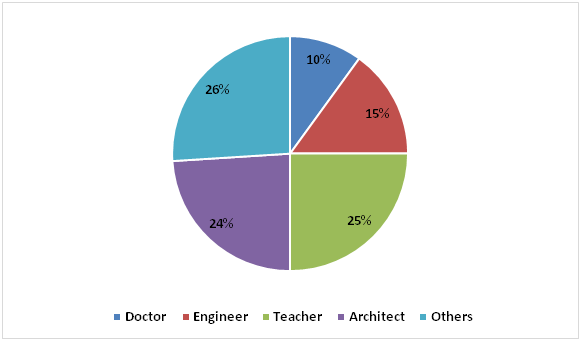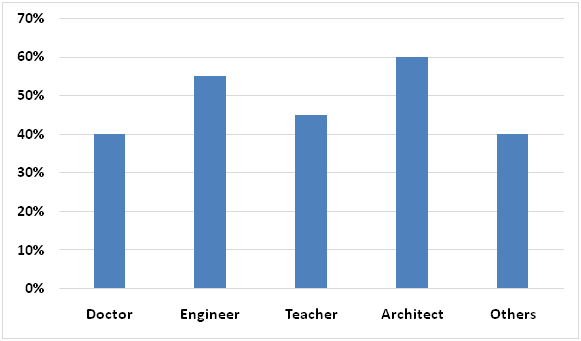# SBI Clerk Pre Quantitative Aptitude (Day-29)

Dear Aspirants, Our IBPS Guide team is providing new series of Quantitative Aptitude Questions for SBI Clerk Prelims 2020 so the aspirants can practice it on a daily basis. These questions are framed by our skilled experts after understanding your needs thoroughly. Aspirants can practice these new series questions daily to familiarize with the exact exam pattern and make your preparation effective.

Start Quiz

Ensure Your Ability Before the Exam – Take SBI Clerk 2020 Prelims Free Mock Test

Data Interpretation

Directions (1 – 5): Study the following information carefully and answer the questions given below?

The following pie chart represents percentage wise distribution of total number of people in a town having different profession.The following bar graph represents percentage of males among them.1) If number of Engineers in the town is 1200, find the respective ratio of number of male doctors and number of female teachers in the town?

a) 7:47

b) 9:49

c) 11:53

d) 16:55

e) None of these

2) If number of male teachers in the town is 1350, find the average of the number of doctors, architects and number of people having other professions in the town.

a) 2400

b) 2000

c) 2500

d) 2800

e) None of these

3) If total number of people in the town is 20000, find the total number of males in the town?

a) 6000

b) 7220

c) 8440

d) 9660

e) None of these

4) Find the number of Carpenters in the town?

Statement I: Number of Carpenters in the town is 10% of the number of people having other professions in the town.

Statement II: Number of male Architects in the town is 3600.

a) If the data in statement I alone are sufficient.

b) If the data in statement II alone are sufficient.

c) If the data either in statement I alone or statement II alone are sufficient to answer.

d) If the data given in both I and II together are not sufficient.

e) If the data in both the statements I and II together are necessary to answer.

5) Find the respective ratio of number of female Tailors in the town and number of female doctors in the town.

Statement I: 35% of the total number of Tailors are females.

Statement II: 15% of the number of people having other profession in the town are Tailors.

a) If the data in statement I alone are sufficient.

b) If the data in statement II alone are sufficient.

c) If the data either in statement I alone or statement II alone are sufficient to answer.

d) If the data given in both I and II together are not sufficient.

e) If the data in both the statements I and II together are necessary to answer.

Directions (6 – 10): In each of the following questions, two equations are given. You have to solve these equations to find the relation between x and y and give answer as,

a) If x < y

b) If x > y

c) If x ≤ y

d) If x ≥ y

e) If relationship between x and y cannot be determined

6) I) 8x2 – 15x + 7 = 0

II) 2y2 – 15y + 28 = 0

7) I) 2x2 – 7x + 6 = 0

II) y2 + 13y + 42 = 0

8) I) 2x2 – 9x + 9 = 0

II) 8y2+ 34y + 21 = 0

9) I) 2x2 – 13x + 15 = 0

II) 3y2 + 28y + 65 = 0

10) I) x2 = 2401

II) y3 = 117649

Directions (1-5) :

Total number of people in the town = 100/15 x 1200 = 8000

Number of male doctors in the town = 40/100 x 10/100 x 8000 = 320

Number of female teachers in the town = (100 – 45)/100 x 25/100 x 8000

= 55/100 x 25/100 x 8000

= 1100

Required ratio = 320: 1100 = 16:55

Let, total number of people in the town = k

45/100 x 25/100 x k = 1350

=> k = 1350 x 100/25 x 100/45

=> k = 12000

Number of doctors in the town = 10/100 x 12000 = 1200

Number of architects in the town = 24/100 x 12000 = 2880

Number of people having other professions in the town = 26/100 x 12000

= 3120

Required average = (1200 + 2880 + 3120)/3

= 7200/3

= 2400

Number of male doctors in the town = 40/100 x 10/100 x 20000 = 800

Number of male Engineers in the town = 55/100 x 15/100 x 20000 = 1650

Number of male Teachers in the town = 45/100 x 25/100 x 20000 = 2250

Number of male Architects in the town = 60/100 x 24/100 x 20000 = 2880

Number of males in the town having other professions

= 40/100 x 26/100 x 20000 = 2080

Required sum = 800 + 1650 + 2250 + 2880 + 2080 = 9660

From I:

Number of Carpenters in the town is 10% of the number of people having other professions in the town.

From II:

Let, total number of people in the town = k

60/100 x 24/100 x k = 3600

=> k = 3600 x 100/24 x 100/60

=> k = 25000

From I and II:

Number of Carpenters in the town = 10/100 x 26/100 x 25000 = 650

Hence, data in both the statements I and II together are necessary to answer.

From I:

35% of the total number of Tailors are females.

From II:

15% of the number of people having other profession in the town are Tailors.

From I and II:

Let, total number of people in the town = n

Number of female Tailors in the town= 35/100 x 15/100 x 26/100 x n

Number of female Doctors in the town = 60/100 x 10/100 x n

Required ratio = 35/100 x 15/100 x 26/100 x n : 60/100 x 10/100 x n

= 91:400

Hence, data in both the statements I and II together are necessary to answer.

Directions (6-10) :

I) 8x2 – 15x + 7 = 0

=> 8x2 – 8x – 7x + 7 = 0

=> 8x(x – 1) – 7(x – 1) = 0

=> (8x – 7)(x – 1) = 0

=> x = 7/8, 1

II) 2y2 – 15y + 28 = 0

=> 2y2 – 8y – 7y + 28 = 0

=> 2y(y – 4) – 7(y – 4) = 0

=> (2y – 7)(y – 4) = 0

=> y = 7/2, 4

Hence, x < y

I) 2x2 – 7x + 6 = 0

=> 2x2 – 4x – 3x + 6 = 0

=> 2x(x – 2) – 3(x – 2) = 0

=> (2x – 3)(x – 2) = 0

=> x = 3/2, 2

II) y2 + 13y + 42 = 0

=> y2 + 6y + 7y + 42 = 0

=> y(y + 6) + 7(y + 6) = 0

=> (y + 7)(y + 6) = 0

=> y = -7, -6

Hence, x > y

I) 2x2– 9x + 9 = 0

=> 2x2 – 6x – 3x + 9 = 0

=> 2x(x – 3) – 3(x – 3) = 0

=> (2x – 3)(x – 3) = 0

=> x = 3/2, 3

II) 8y2+ 34y + 21 = 0

=> 8y2 + 28y + 6y + 21 = 0

=> 4y(2y + 7) + 3(2y + 7) = 0

=> (4y + 3)(2y + 7) = 0

=> y = -3/4, -7/2

Hence, x > y

I) 2x2 – 13x + 15 = 0

=> 2x2 – 10x – 3x + 15 = 0

=> 2x(x – 5) – 3(x – 5) = 0

=> (2x – 3)(x – 5) = 0

=> x = 3/2, 5

II) 3y2 + 28y + 65 = 0

=> 3y2 + 15y + 13y + 65 = 0

=> 3y(y + 5) + 13(y + 5) = 0

=> (3y + 13)(y + 5) = 0

=> y = -13/3, -5

Hence, x > y

I) x2 = 2401

=> x = ± √2401

=> x = ± 49

II) y3 = 117649

=> y = ∛117649

=> y = 49

Hence, x ≤ y

 Check Here to View SBI Clerk Prelims 2020 Quantitative Aptitude Questions Day 28 Day 27 Day 26 Click Here for SBI Clerk 2020 – Detailed Exam Notification